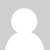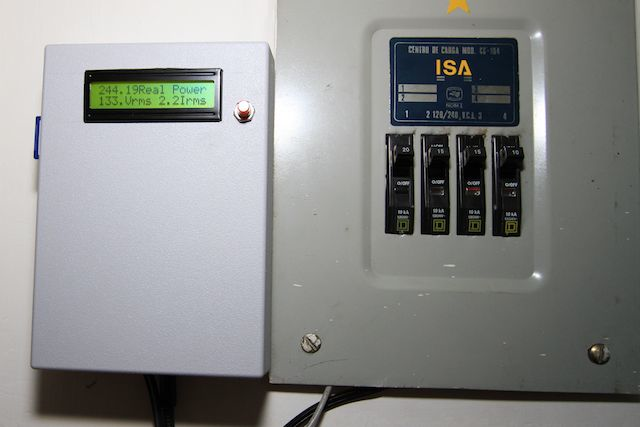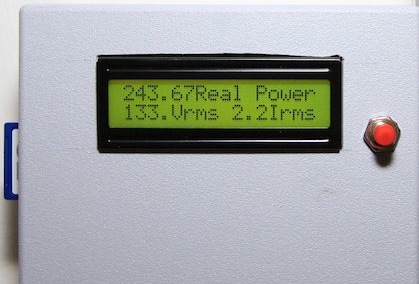### power monitor with LCD and SD for information storage

Hi

I just wanted to post the code for my Energy Monitor, it is based on Trystan Lea and Eric Sandeen code. i just added a LCD 16x2 menu and a way to storage data in a SD card. For this I used Adafruit Loggin shield, http://www.adafruit.com/products/243 and seedstudio 100 Amp CT. I hope its useful, if you need more informatin please ask. Thanks a lot for your support, this project was very fun to do and I hope to keep evolving it, maybe next phase will be to stream the information to a web server installed in a modiffied apple tv 1 (the only computer that is on all the time at home)

//Basic energy monitoring sketch
//Based on design by: Trystan Lea, Eric Sandeen
//Licenced under GNU General Public Licence
// openenergymonitor.org
// Patricio Duenas

//Sketch measures voltage and current.
//and then calculates useful values like real power,
//apparent power, powerfactor, Vrms, Irms.

// Connections:
// rs (LCD pin 4) to Arduino pin 9
// rw (LCD pin 5) to Arduino pin 8
// enable (LCD pin 6) to Arduino pin 7
// Arduino pin 6 goes to pushbutton for menu
// LCD pins d4, d5, d6, d7 to Arduino pins 5, 4, 3, 2

#include <LiquidCrystal.h>
#include <Wire.h>
#include "RTClib.h"
#include <SD.h>

//initialize lcd
LiquidCrystal lcd(9, 8, 7, 5, 4, 3, 2);

//Setup variables
int numberOfSamples = 3000;

//Set Voltage and current input pins
int inPinV = 2;
int inPinI = 1;

//define RTC object
RTC_DS1307 RTC;

//Sanity check calibration method thanks to Eric Sandeen http://sandeen.net/wordpress
//See discussion here: http://openenergymonitor.org/emon/node/59
//Enter the values of your setup below

// Voltage is reduced both by wall xfmr & voltage divider
#define AC_WALL_VOLTAGE        130
// Ratio of the voltage divider in the circuit
#define AC_VOLTAGE_DIV_RATIO   3.3859
// CT: Voltage depends on current, burden resistor, and turns
#define CT_BURDEN_RESISTOR    88
#define CT_TURNS              3030

//Calibration coeficients
//These need to be set in order to obtain accurate results
//Set the above values first and then calibrate futher using normal calibration method described on how to build it page.
double VCAL = 1.111;
double ICAL = 0.62;
double PHASECAL = 2;

// Initial gueses for ratios, modified by VCAL/ICAL tweaks
double V_RATIO = AC_ADAPTER_RATIO * AC_VOLTAGE_DIV_RATIO * 5 / 1024 * VCAL;
double I_RATIO = (long double)CT_TURNS / CT_BURDEN_RESISTOR * 5 / 1024 * ICAL;

//Sample variables
int lastSampleV,lastSampleI,sampleV,sampleI;

//Filter variables
double lastFilteredV, lastFilteredI, filteredV, filteredI;
double filterTemp;

//Stores the phase calibrated instantaneous voltage.
double shiftedV;

//Power calculation variables
double sqI,sqV,instP,sumI,sumV,sumP;

//Useful value variables
double realPower,
apparentPower,
powerFactor,
Vrms,
Irms;

//SD writing related variables
const int chipSelect = 10;   //SD CS for adafruit shield
File logfile;   // the logging file
#define SYNC_INTERVAL 60000   //interval to write data to SD, here is set to 1 munite in miliseconds
//minus 1 sec for the V and I sampling time
uint32_t syncTime = 0;  //time of last sync

void setup()
{
Serial.begin(57600);
Wire.begin();
RTC.begin();
RTC.adjust(DateTime(__DATE__, __TIME__));  //set RTC to computer's clock at the time of compilation

// make sure that the default chip select pin is set to
// output, even if you don't use it:
pinMode(10, OUTPUT);
// see if the card is present and can be initialized:
lcd.begin(16,2);
lcd.clear();
lcd.setCursor(0,0);
if (!SD.begin(chipSelect)) {
lcd.print("Card failed, or");
lcd.setCursor(0,1);
lcd.print("not present");
delay(5000);
lcd.clear();
}else{
lcd.print("card initialized");
delay(2000);
// create a new file
char filename[] = "LOGGER00.CSV";          //Every time the Arduino starts, we will create
for (uint8_t i = 0; i < 100; i++) {        //a new file named LOGGERnn.csv
filename = i/10 + '0';
filename = i%10 + '0';
if (! SD.exists(filename)) {
// only open a new file if it doesn't exist
logfile = SD.open(filename, FILE_WRITE);
break;  // leave the loop!
}
}
logfile.println("date,time,realPower,apparentPower,powerFactor,Vrms,Irms");  //this is the header of the csv file
}
}

void loop()
{
if ( menusum == HIGH) {
}
for (int n=0; n<numberOfSamples; n++)
{
//Used for offset removal
lastSampleV=sampleV;
lastSampleI=sampleI;

//Read in voltage and current samples.

//Used for offset removal
lastFilteredV = filteredV;
lastFilteredI = filteredI;

//Digital high pass filters to remove 2.5V DC offset.
filteredV = 0.996*(lastFilteredV+sampleV-lastSampleV);
filteredI = 0.996*(lastFilteredI+sampleI-lastSampleI);

//Phase calibration goes here.
shiftedV = lastFilteredV + PHASECAL * (filteredV - lastFilteredV);

//Root-mean-square method voltage
//1) square voltage values
sqV= filteredV * filteredV;
//2) sum
sumV += sqV;

//Root-mean-square method current
//1) square current values
sqI = filteredI * filteredI;
//2) sum
sumI += sqI;

//Instantaneous Power
instP = shiftedV * filteredI;
//Sum
sumP +=instP;
}

//Calculation of the root of the mean of the voltage and current squared (rms)
//Calibration coeficients applied.
Vrms = V_RATIO*sqrt(sumV / numberOfSamples);
Irms = I_RATIO*sqrt(sumI / numberOfSamples);

//Calculation power values
realPower = V_RATIO*I_RATIO*sumP / numberOfSamples;
apparentPower = Vrms * Irms;
powerFactor = realPower / apparentPower;

//output to serial  i, v, real p
Serial.print(Vrms);
Serial.print("  ");
Serial.print(Irms);
Serial.print("  ");
Serial.println(realPower);

//output to lcd
DateTime now = RTC.now();

lcd.setCursor(4,0);           // set cursor to column 0, row 0 (the first row)
lcd.print(now.day(), DEC);
lcd.print("/");
lcd.print(now.month(), DEC);
lcd.print("/");
lcd.print(now.year(), DEC);
lcd.setCursor(5,1);
lcd.print(now.hour(), DEC);
lcd.print(":");
if(now.minute() < 10){   //little trick to print 0 to 9 minutes
lcd.print("0");
}
lcd.print(now.minute(), DEC);
}

lcd.setCursor(0,0);           // set cursor to column 0, row 0 (the first row)
lcd.print(realPower);
lcd.setCursor(6,0);           //main menu, will have to change to a more complex one
lcd.print("Real Power");
lcd.setCursor(0,1);
lcd.print(Vrms);
lcd.setCursor(4,1);
lcd.print("Vrms");
lcd.setCursor(9,1);
lcd.print(Irms);
lcd.setCursor(12,1);
lcd.print("Irms");
}

lcd.setCursor(0,0);           // set cursor to column 0, row 0 (the first row)
lcd.print(powerFactor);
lcd.setCursor(6,0);           //main menu, will have to change to a more complex one
lcd.print("Pwr Factor");
lcd.setCursor(0,1);
lcd.print(apparentPower);
lcd.setCursor(7,1);
lcd.print("Aprnt Pwr");
}

}

//Reset accumulators
sumV = 0;
sumI = 0;
sumP = 0;

//Save data to disk once every minute
if ((millis() - syncTime) < SYNC_INTERVAL) return;
syncTime = millis();

//write data to SD
logfile.print(now.year(), DEC);
logfile.print("/");
logfile.print(now.month(), DEC);
logfile.print("/");
logfile.print(now.day(), DEC);
logfile.print(" ");
logfile.print(",");
logfile.print(now.hour(), DEC);
logfile.print(":");
logfile.print(now.minute(), DEC);
logfile.print(":");
logfile.print(now.second(), DEC);
logfile.print(", ");
logfile.print(realPower);
logfile.print(", ");
logfile.print(apparentPower);
logfile.print(", ");
logfile.print(powerFactor);
logfile.print(", ");
logfile.print(Vrms);
logfile.print(", ");
logfile.println(Irms);
logfile.flush();

}

### Re: power monitor with LCD and SD for information storage

Hi Mexican Watt,

Thanks for posting your code. I am sure people will find it very useful. It would be really cool if you could post a few photos of your setup. It would bring the code alive!

All the best, Glyn.### Re: power monitor with LCD and SD for information storage

Hi, Sorry for my late response, here are some photos of the exterior. I will post some of the interesting part, the circuitry when I reopen the box for some troubleshooting with the clock unit### Re: power monitor with LCD and SD for information storage

That looks really neat, nice work!